# fill3

Create filled 3-D patches

•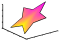## Syntax

``fill3(X,Y,Z,C)``
``fill3(X1,Y1,Z1,C1,...,Xn,Yn,Zn,Cn)``
``fill3(___,Name,Value)``
``fill3(ax,___)``
``p = fill3(___)``

## Description

example

````fill3(X,Y,Z,C)` plots filled polygonal regions on 3-D axes as `Patch` objects with vertices at the (x,y,z) locations specified by `X`, `Y`, and `Z`. To plot one region, specify `X`, `Y`, and `Z` as vectors.To plot multiple regions, specify `X`, `Y`, and `Z` as matrices where each column corresponds to a polygon. `C` determines the fill colors for the regions.```

example

````fill3(X1,Y1,Z1,C1,...,Xn,Yn,Zn,Cn)` plots multiple filled polygonal regions on the same 3-D axes. ```
````fill3(___,Name,Value)` modifies the `Patch` objects using one or more name-value arguments to set properties. Patches can be specified using any of the input argument combinations in previous syntaxes. For example, `fill3(X,Y,Z,C,'LineWidth',2)` specifies a two-point border around all the patches. For a list of properties, see Patch Properties.```
````fill3(ax,___)` plots the polygonal regions in the axes specified by `ax` instead of in the current axes (`gca`). ```

example

````p = fill3(___)` returns a `Patch` object or a vector of `Patch` objects. Use `p` to query and modify properties after plotting a region. For a full list of properties, see Patch Properties.```

## Examples

collapse all

Specify vectors `x`, `y`, and `z` as the (x,y,z) coordinates of the vertices for a triangle. Then plot the triangle with the specified fill color, red.

```x = [0 0 0]; y = [0 2 1]; z = [0 0 2]; fill3(x,y,z,'r')```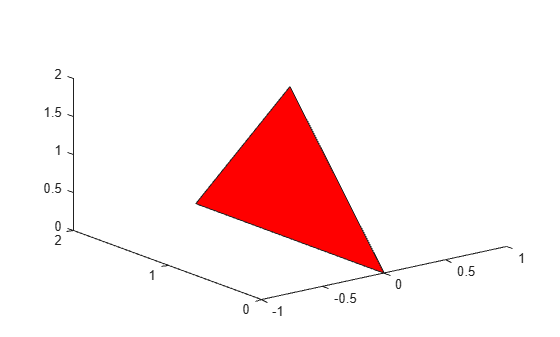Define vectors `x1`, `y1`, `z1` and `x2`, `y2`, `z2` as the (x,y,z) coordinates of the vertices for two rectangles. Specify the color of each rectangle as an RGB triplet, with values dictating the intensities of the red, green, and blue components of the color. Plot both rectangles in a single `fill3` call.

```x1 = [0 0 1 1]; y1 = [3 3 2 2]; z1 = [0 3 2 1]; c1 = [0 0.447 0.741]; x2 = [2 2 3 3]; y2 = [1 1 0 0]; z2 = [1 2 3 0]; c2 = [0.850 0.325 0.098]; fill3(x1,y1,z1,c1,x2,y2,z2,c2)```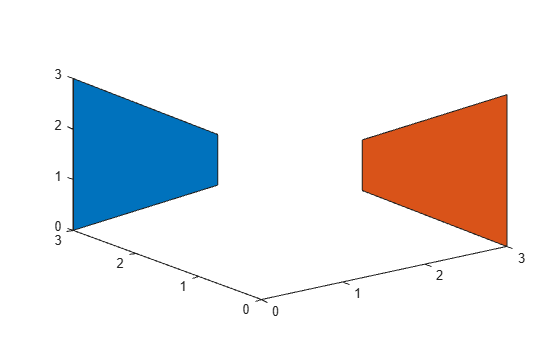Specify matrices `x`, `y`, and `z` as the coordinates of the vertices for three adjacent squares. Specify `c` as a matrix of the same dimensions as `x`, `y`, and `z`. Each value in `c` specifies a colormap index for the corresponding vertex. The `fill3` function interpolates the fill color of each square from the vertex colors.

```x = [0 0 0; 0 0 2; 0 2 2; 0 2 0]; y = [2 0 2; 0 0 2; 0 0 0; 2 0 0]; z = [2 0 2; 2 2 2; 0 2 2; 0 0 2]; c = [2 2 2; 3 3 0; 2 2 2; 0 0 3]; fill3(x,y,z,c)```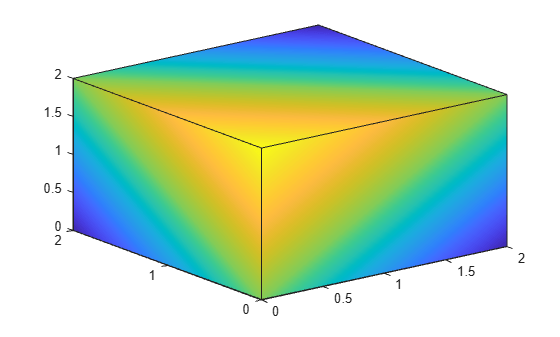Plot two triangles with fill colors specified by vector `c` of colormap indices. Store the two returned patches in vector `p`.

```x = [0 1; 1.5 2.5; 3 4]; y = [4 4; 2.5 2.5; 1 1]; z = [0 0; 2 2; 0 0]; c = [1 0]; p = fill3(x,y,z,c);```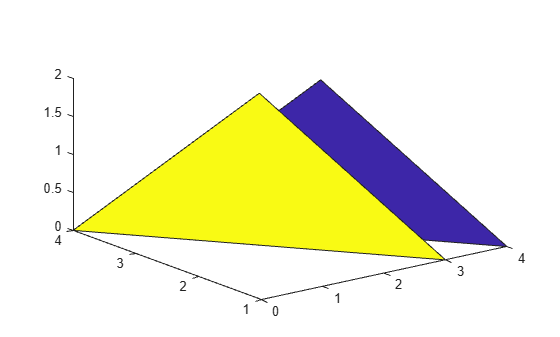Use `p` to modify the first triangle. Modify the `FaceAlpha` property of the first element of `p` to make the first triangle transparent.

`p(1).FaceAlpha = 0.5;`## Input Arguments

collapse all

x-coordinates for the vertices, specified in one of these forms:

• Vector — Create one polygonal region.

• Matrix — Create n polygonal regions with m vertices each, where `X` is an m-by-n matrix. Each column in the matrix corresponds to one polygonal region.

If `X`, `Y`, or `Z` is a matrix, `fill3` replicates any column vector arguments to produce matrices of the required size.

If the data does not define closed regions, then `fill3` closes the regions.

Data Types: `single` | `double` | `int8` | `int16` | `int32` | `int64` | `uint8` | `uint16` | `uint32` | `uint64` | `categorical` | `datetime` | `duration`

y-coordinates for the vertices, specified in one of these forms:

• Vector — Create one polygonal region.

• Matrix — Create n polygonal regions with m vertices each, where `Y` is an m-by-n matrix. Each column in the matrix corresponds to one polygonal region.

If `X`, `Y`, or `Z` is a matrix, `fill3` replicates any column vector arguments to produce matrices of the required size.

If the data does not define closed regions, then `fill3` closes the regions.

Data Types: `single` | `double` | `int8` | `int16` | `int32` | `int64` | `uint8` | `uint16` | `uint32` | `uint64` | `categorical` | `datetime` | `duration`

z-coordinates for the vertices, specified in one of these forms:

• Vector — Create one polygonal region.

• Matrix — Create n polygonal regions with m vertices each, where `Z` is an m-by-n matrix. Each column in the matrix corresponds to one polygonal region.

If `X`, `Y`, or `Z` is a matrix, `fill3` replicates any column vector arguments to produce matrices of the required size.

If the data does not define closed regions, then `fill3` closes the regions.

Data Types: `single` | `double` | `int8` | `int16` | `int32` | `int64` | `uint8` | `uint16` | `uint32` | `uint64` | `categorical` | `datetime` | `duration`

Patch colors, specified as a color name, RGB triplet, vector of colormap indices, or a matrix of colormap indices.

• Color name — A color name such as `'red'`, or a short name such as `'r'`.

• RGB triplet — A three-element row vector whose elements specify the intensities of the red, green, and blue components of the color. The intensities must be in the range `[0,1]`; for example, ```[0.4 0.6 0.7]```. RGB triplets are useful for creating custom colors.

• Vector of colormap indices — A vector of numeric values that has one element for each region.

• Matrix of colormap indices — A matrix of numeric values that has the same dimensions as `X`, `Y`, and `Z`.

The way you specify the color depends on the color scheme and whether you are plotting one polygonal region or multiple regions. This table describes the most common situations.

Color SchemeHow to Specify the ColorExample
Single color for all regions

Specify a color name or a short name from the table below, or specify one RGB triplet.

Create matrices `x`, `y`, and `z`. Then plot the filled region in red.

```x = [0.5 0; 0.5 0; 1 1]; y = [0 2; 2 6; 1 4]; z = [0 0; 0 0; 1 2]; fill3(x,y,z,'r')```One color per region

Specify an n-by-1 or 1-by-n vector of colormap indices, where `n` is the number of polygonal regions.

Create matrices `x`, `y`, and `z` and vector `c`. Then plot the filled region in the specified colormap colors.

```x = [0.5 0; 0.5 0; 1 1]; y = [0 2; 2 6; 1 4]; z = [0 0; 0 0; 1 2]; c = [1 0]; fill3(x,y,z,c)```Interpolated face colors

Specify an m-by-n matrix of colormap indices, where `[m,n] = size(X)`. Specify one color per vertex.

Create matrices `x`, `y`, `z` and `c`. Then plot the filled region with fill color interpolated from vertex colors `c`.

```x = [0.5 0; 0.5 0; 1 1]; y = [0 2; 2 6; 1 4]; z = [0 0; 0 0; 1 2]; c = [1 1; 1 0.5; 0 0]; fill3(x,y,z,c)```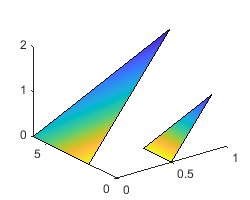#### Color Names and RGB Triplets for Common Colors

Color NameShort NameRGB TripletHexadecimal Color CodeAppearance
`'red'``'r'``[1 0 0]``'#FF0000'``'green'``'g'``[0 1 0]``'#00FF00'``'blue'``'b'``[0 0 1]``'#0000FF'``'cyan'` `'c'``[0 1 1]``'#00FFFF'``'magenta'``'m'``[1 0 1]``'#FF00FF'``'yellow'``'y'``[1 1 0]``'#FFFF00'``'black'``'k'``[0 0 0]``'#000000'``'white'``'w'``[1 1 1]``'#FFFFFF'`Here are the RGB triplets and hexadecimal color codes for the default colors MATLAB® uses in many types of plots.

`[0 0.4470 0.7410]``'#0072BD'``[0.8500 0.3250 0.0980]``'#D95319'``[0.9290 0.6940 0.1250]``'#EDB120'``[0.4940 0.1840 0.5560]``'#7E2F8E'``[0.4660 0.6740 0.1880]``'#77AC30'``[0.3010 0.7450 0.9330]``'#4DBEEE'``[0.6350 0.0780 0.1840]``'#A2142F'`Target axes, specified as an `Axes` object. If you do not specify the axes, the `fill3` function plots into the current axes or creates an `Axes` object if one does not exist.

### Name-Value Arguments

Specify optional pairs of arguments as `Name1=Value1,...,NameN=ValueN`, where `Name` is the argument name and `Value` is the corresponding value. Name-value arguments must appear after other arguments, but the order of the pairs does not matter.

Before R2021a, use commas to separate each name and value, and enclose `Name` in quotes.

Example: `fill3(x,y,z,c,'FaceAlpha',.5,'LineStyle',':')` creates semitransparent polygons with dotted edges.

Note

The properties listed here are only a subset of patch properties. For a complete list, see Patch Properties.

Face color, specified as `'interp'`, `'flat'` an RGB triplet, a hexadecimal color code, a color name, or a short name.

To create a different color for each face, specify the `CData` or `FaceVertexCData` property as an array containing one color per face or one color per vertex. The colors can be interpolated from the colors of the surrounding vertices of each face, or they can be uniform. For interpolated colors, specify this property as `'interp'`. For uniform colors, specify this property as `'flat'`. If you specify `'flat'` and a different color for each vertex, the color of the first vertex you specify determines the face color.

To designate a single color for all of the faces, specify this property as an RGB triplet, a hexadecimal color code, a color name, or a short name.

• An RGB triplet is a three-element row vector whose elements specify the intensities of the red, green, and blue components of the color. The intensities must be in the range `[0,1]`; for example, `[0.4 0.6 0.7]`.

• A hexadecimal color code is a character vector or a string scalar that starts with a hash symbol (`#`) followed by three or six hexadecimal digits, which can range from `0` to `F`. The values are not case sensitive. Thus, the color codes `'#FF8800'`, `'#ff8800'`, `'#F80'`, and `'#f80'` are equivalent.

Color NameShort NameRGB TripletHexadecimal Color CodeAppearance
`'red'``'r'``[1 0 0]``'#FF0000'``'green'``'g'``[0 1 0]``'#00FF00'``'blue'``'b'``[0 0 1]``'#0000FF'``'cyan'` `'c'``[0 1 1]``'#00FFFF'``'magenta'``'m'``[1 0 1]``'#FF00FF'``'yellow'``'y'``[1 1 0]``'#FFFF00'``'black'``'k'``[0 0 0]``'#000000'``'white'``'w'``[1 1 1]``'#FFFFFF'``'none'`Not applicableNot applicableNot applicableNo color

Here are the RGB triplets and hexadecimal color codes for the default colors MATLAB uses in many types of plots.

`[0 0.4470 0.7410]``'#0072BD'``[0.8500 0.3250 0.0980]``'#D95319'``[0.9290 0.6940 0.1250]``'#EDB120'``[0.4940 0.1840 0.5560]``'#7E2F8E'``[0.4660 0.6740 0.1880]``'#77AC30'``[0.3010 0.7450 0.9330]``'#4DBEEE'``[0.6350 0.0780 0.1840]``'#A2142F'`Face transparency, specified as one of these values:

• Scalar in range `[0,1]` — Use uniform transparency across all of the faces. A value of `1` is fully opaque and `0` is completely transparent. This option does not use the transparency values in the `FaceVertexAlphaData` property.

• `'flat'` — Use a different transparency for each face based on the values in the `FaceVertexAlphaData` property. First you must specify the `FaceVertexAlphaData` property as a vector containing one transparency value per face or vertex. The transparency value at the first vertex determines the transparency for the entire face.

• `'interp'` — Use interpolated transparency for each face based on the values in `FaceVertexAlphaData` property. First you must specify the `FaceVertexAlphaData` property as a vector containing one transparency value per vertex. The transparency varies across each face by interpolating the values at the vertices.

Edge colors, specified as one of the values in this table. The default edge color is black with a value of `[0 0 0]`. If multiple polygons share an edge, then the first polygon drawn controls the displayed edge color.

ValueDescriptionResult

RGB triplet, hexadecimal color code, or color name

Single color for all of the edges. See the following table for more details.`'flat'`

Different color for each edge. Use the vertex colors to set the color of the edge that follows it. You must first specify `CData` or `FaceVertexCData` as an array containing one color per vertex. The edge color depends on the order in which you specify the vertices.`'interp'`

Interpolated edge color. You must first specify `CData` or `FaceVertexCData` as an array containing one color per vertex. Determine the edge color by linearly interpolating the values at the two bounding vertices.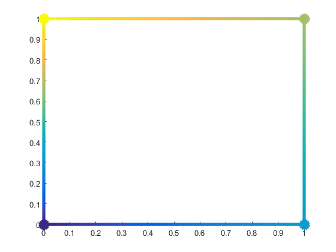`'none'`No edges displayed.

No edges displayed.

RGB triplets and hexadecimal color codes are useful for specifying custom colors.

• An RGB triplet is a three-element row vector whose elements specify the intensities of the red, green, and blue components of the color. The intensities must be in the range `[0,1]`; for example,``` [0.4 0.6 0.7]```.

• A hexadecimal color code is a character vector or a string scalar that starts with a hash symbol (`#`) followed by three or six hexadecimal digits, which can range from `0` to `F`. The values are not case sensitive. Thus, the color codes `'#FF8800'`, `'#ff8800'`, `'#F80'`, and `'#f80'` are equivalent.

Alternatively, you can specify some common colors by name. This table lists the named color options, the equivalent RGB triplets, and hexadecimal color codes.

Color NameShort NameRGB TripletHexadecimal Color CodeAppearance
`'red'``'r'``[1 0 0]``'#FF0000'``'green'``'g'``[0 1 0]``'#00FF00'``'blue'``'b'``[0 0 1]``'#0000FF'``'cyan'` `'c'``[0 1 1]``'#00FFFF'``'magenta'``'m'``[1 0 1]``'#FF00FF'``'yellow'``'y'``[1 1 0]``'#FFFF00'``'black'``'k'``[0 0 0]``'#000000'``'white'``'w'``[1 1 1]``'#FFFFFF'`Here are the RGB triplets and hexadecimal color codes for the default colors MATLAB uses in many types of plots.

`[0 0.4470 0.7410]``'#0072BD'``[0.8500 0.3250 0.0980]``'#D95319'``[0.9290 0.6940 0.1250]``'#EDB120'``[0.4940 0.1840 0.5560]``'#7E2F8E'``[0.4660 0.6740 0.1880]``'#77AC30'``[0.3010 0.7450 0.9330]``'#4DBEEE'``[0.6350 0.0780 0.1840]``'#A2142F'`Line style, specified as one of the options listed in this table.

Line StyleDescriptionResulting Line
`'-'`Solid line`'--'`Dashed line`':'`Dotted line`'-.'`Dash-dotted line`'none'`No lineNo line

## Output Arguments

collapse all

Displayed polygonal regions, returned as a `Patch` object or vector of `Patch` objects. Each patch corresponds to a plotted region. Use `p` to query or change properties of a region after it is plotted.

## Alternative Functionality

Use the `patch` function to create filled polygons on 3-D axes. This function provides several additional options for defining and configuring filled regions:

• Create polygons by specifying a set of faces and vertices.

• Specify multiple n-gons of varying n within the same matrices.

• Interpolate face colors from custom RBG values.

## Version History

Introduced before R2006a

expand all

Behavior changed in R2021a